# IBPS SO Exam 2015: Quantitative Aptitude-Concept and Sample Question-Averages

The banking team of jagranjosh.com has come up with concept and sample questions for Averages. The concept provided by us will help you to understand the topic. The sample questions offered by us are framed by keeping in view need of the question paper.

The banking team of jagranjosh.com has come up with concept and sample questions for Averages. The concept provided by us will help you to understand the topic. The sample questions offered by us are framed by keeping in view need of the question paper.

1. The term “Average” conventionally implies "Arithmetic mean". The arithmetic mean is obtained by dividing the sum of all the numbers (of the group) by the total number. In terms of formula we get,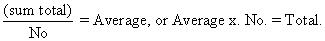2. For any two positive numbers "a" and "b", we have

(A) Arithmetic Mean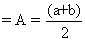..... (Popularly known as A.M.)

(B) Geometric Mean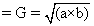……. (Popularly known as G.M.)

(C) Harmonic Mean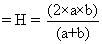…….. (Popularly known as H.M.)

Further, G2=AM.GM = (A) (H) AND H.M ≤ G.M. ≤ A.M

3. The concept of "Harmonic Mean" is applicable in cases where a motorcar (for example) travels from A to B (a fixed distance) at the speed of, say, "X" kmph. and returns from B to A (the same distance) at the speed of, say, “Y" kmph. and we are interested to know the overall speed of the entire journey. This overall speed is obtained by the application of the formula of Harmonic Mean (H.M.) as follows:

required overall speed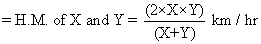4. Weighted Average: If the weights of numbers x1,x2,x3,x4…….. etc. are W1 , W2 , W3, W4……. etc respectively, then, the weighted average of these numbers is given as: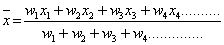(In case of simple arithmetic average, the weights are equal Thus, we have, W1 = w2 = W3 = W4 ..... = 1 each).

Sample Questions